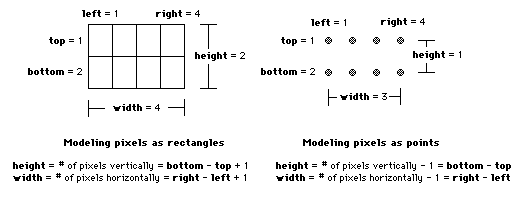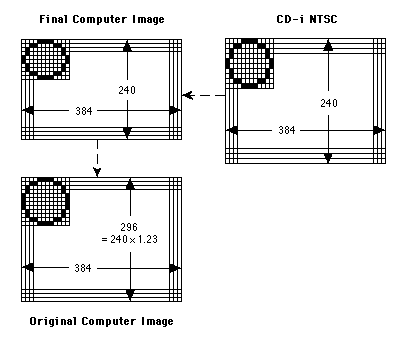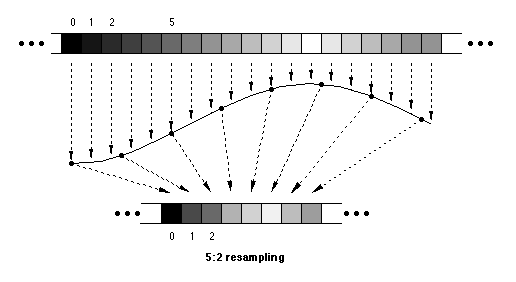# Aspect Ratios:A Frank Yet Candid Discussion

## INTRODUCTION

The whole question of aspect ratios is filled with misunderstandings, many of them "just" semantic, but of course whatever the cause, the result is confusion. First, some definitions.

```    quantity                         definition                     symbol
========          =========================================   ===========
physical dimensions   Height and width of screen image in cm.      ScreenH,W
logical dimensions      "     "    "    "    "     "    " pixels   PixelsX,Y
pixel pitch            Measured in cm/pixel.                       PixelPitchX,Y

```
(Could use dots-per-cm as the reciprocal of pitch.)

Now the undisputed physical fact (length of an object) is:

```            ScreenW  =  PixelPitchX × PixelsX                     [Eq. 1]
(cm)       (cm/pix)      (pix)

```
(and likewise in the y-direction). Taking the ratio of y to x:

```              ScreenH         PixelPitchY     PixelsY
———————    =    ———————————  ×  ———————             [Eq. 2]
ScreenW         PixelPitchX     PixelsX

physical         pixel         logical
(display)	       (aspect)      (data)
ratio            ratio          ratio
(often 0.75)

```
All three of these ratios are called the aspect ratio in various contexts! Let's keep them separate. Hereafter we will drop the word "aspect" from the term "pixel ratio".

Note that one may model pixels as points or rectangles. If they are points, then what we call "pixel size" should properly be called "pixel spacing." In addition, Eq. 1 should read

`             Screen = PixelSpacing × (Pixels - 1)`

since the image does not extend to the left of the first pixel or to the right of the last. (See Fig. 1)### Figure 1

In this document we will be thinking of pixels as rectangles with non-zero height and width. The alteration needed, for pixels as points, in subsequent equations is trivial, and in fact the difference it makes becomes negligible for large images.

## IMAGE CONVERSION

Differing pixel ratios have to be accounted for when transferring artwork from one medium to another. First consider what will happen if an image is generated on a computer and then transferred pixel-for-pixel directly to CD-i. Computer displays typically have a pixel ratio close to 1. The NTSC CD-i pixels are taller, with a ratio of 1.23. Figure 2 shows what will happen if no image correction is applied: what was a circle to the artist at the computer screen is a tall ellipse to the CD-i viewer.### Figure 2

How do we arrive at a good-looking CD-i display? Before we develop a general equation, we can work backward from the desired result to the starting image that will yield that result. In Figure 3, we have a CD-i display with a circle that looks properly round. (Artwork limitations make it look a bit tall. Consider it round.)### Figure 3

Moving back to the computer image that generates it, we see that the "circle" must look short. In fact, everything in the artwork must look vertically squashed. Well, this is not a convenient way for artists to work. How can the artist work in a domain where things look to his or her eye the way they will appear on the final CD-i display? This is where resampling comes in.

The original artwork can be created in a taller size and then squeezed to the proper size. In the process of squeezing, the image will get pre-distorted in such a way that it will look right again when displayed on the CD-i TV screen. The logical height of the original image should be the height of the final image multiplied by the pixel ratio of the final image. (The alert reader will note that the product has been rounded up to an even number, out of sheer obsessive-compulsion.) The general equation that gives the required starting height is Eq. 3b below.

How is this squeezing accomplished? Resampling can be described as the process of reconstructing a smooth curve that describes the variation of brightness & color from pixel to pixel and then finding values on that curve in between the original ones. This is illustrated in the figure below.### Figure 4

It is important to recognize that resampling and pixel ratio change are identical. One cannot resample an image (say to convert from a logical size of 200x100 to a logical size of 100x200) and then do aspect ratio correction to make circles look round again. Once you have resampled, the only remaining factor that bears on the proportion of the final image displayed is the pixel ratio of the playback device.

For example, imagine somebody asks us to convert a 6x4 image into a 12x4 image. We may do this by sampling the source image at twice the resolution horizontally, as shown in Fig. 5.### Figure 5

We obtain the desired 12-horizontal-pixel format, but when the image is displayed, the colored square has become elongated. Resampling has caused/is identical with pixel ratio distortion. To fix this we would have to change the pixel ratio of the destination display, or grab from a shorter rectangle in the source image, or display to a narrower rectangle in the destination display. In the real world the first option will not be available. The second option involves cropping some of the original image - which may not be acceptable, for example in a feature film. The third option will not use all the available display area ("letterboxing").

## THE GENERAL EQUATION

Without clearly defined terminology this is almost impossible to discuss. Defining the terms:

```quantity                      definition                       symbol
========                      ==========                       ======
source pixel ratio            Ratio of pixel size dy/dx          Rs

source logical grab rect      Height & width in pixels of       GrabH,W
region to be resampled

result logical rect           Height & width in pixels of      ResultH,W
resampled image

playback pixel ratio          Pixel ratio on playback device     Rp

pixel ratio distortion        > 1 if playback is too skinny;     D
< 1 if too fat.

```

Consider what the physical display dimensions of the grab rectangle are. From Eq. 2 the ratio of its height to width must be

```                                              GrabH
grab rect physical ratio  =  Rs ×  —————.        [Eq. 3a]
GrabW

```
This rectangle may have to be squeezed or stretched (resampled) to make it fit into the playback rectangle, whose proportion is
```                                             ResultH
playback rect physical ratio  =  Rp × ———————.       [Eq. 3b]
ResultW

```
It is the ratio of these two expressions that gives us the general expression for the distortion of the image:
```                     Rs ×   GrabH/GrabW
D  =  ————————————————————.                  [Eq. 3c]
Rp × ResultH/ResultW

```
If numerator and denominator are equal, a figure that looked square when the original material was played back on the source hardware will still look square on the playback hardware; circles will not turn into ovals, etc. Thus to convert an image from a source pixel ratio of Rs to display properly on a device with pixel ratio Rp, we set D = 1, yielding
```                     GrabH          ResultH
Rs ×  —————  =  Rp × ———————                 [Eq. 4a]
GrabW          ResultW

```
which is the same as
```                          Rp   ResultH
GrabH  =  —— × ——————— × GrabW              [Eq. 4b]
Rs   ResultW

```
which is the same as
```                             GrabH/GrabW
Rp  =  Rs × ———————————————.                 [Eq. 4c]
ResultH/ResultW

```
Notice that Eq. 4b gives the starting (grab) height of an image to be converted as illustrated in Fig. 3 and described above. Eq. 4c is often described by saying that the pixel ratio is being converted from Rs to Rp. The distortion D of this conversion (just the ratio of Eq. 3a and 3b) is given above. Consider, though, that the expression for D is a ratio of ratios times ratios. Discussions of the value or meaning of D usually flounder in misunderstanding.

## PIXEL RATIOS FOR CD-i BASE CASE

CD-i pixel ratios are determined by the video hardware in the CD-i player. The Green Book says (§ V.2.4.1):

```                pixel height
pixel ratio  =  ————————————
pixel width

pixel clock frequency * scan line period   3
=  ———————————————————————————————————————— × —   [Eq. 5]
number of active lines            4

```
How do we understand this in terms of the foregoing? What's the 3/4 all about? Observe that
```PixelsX  =  pixel clock frequency × scan line period (approximately)

PixelsY  =  number of active lines (approximately)
```
Substituting that (and pretending the approximate equalities are exact) yields
```                       PixelsY   3
pixel ratio  =  ——————— × —
PixelsY   4

```
or

```            3/4  =  pixel ratio × logical ratio
```
This is the same as Eq. 2. Thus Eq. 5 says that the CD-i player draws a picture on the screen whose proportions are 3:4 in all cases.

The equalities are not exact for reasons unknown to this author. The Green Book says to use 242.5 as the active line count for NTSC and 287.5 for PAL, instead of 240 and 280 respectively. Similarly, the product of pixel clock rate and active scan line period gives a pixel count larger than 384, or its high-res double value of 768.

To further confuse the issue, the numbers for pixel clock, scan line period, adn pixel ratio in § V.2.4 of the Green Book are incorrect. The correct numbers are:

```format  pixel clock freq  scan line period   pixel ratio
======  ================  ================   ===========
525 TV    15.1049 MHz        52.6555 µ s        1.230	[Green Book: 1.19]
625       15.0 MHz           52.0 µ s           1.017	[Green Book: 1.05]

```
We have empirically measured the NTSC ratio to be 1.235 ± 0.003 and the PAL/SECAM ratio to be 1.019 ± 0.003.

## PIXEL RATIOS FOR DV

The Green Book DV extension discusses the proper pixel ratios for playback of MPEG video in § IX.4.3.2.3. "Full Motion decoders do not compensate for aspect ratio distortion." This means that the decoder does not in fact use the information in the aspect ratio field of the MPEG Video Sequence Header. Thus the following sentence, "It is recommended therefore to select one of the following aspect ratio options: ...", should mean:

Before encoding, correct the (pixel) aspect ratio of the digital image to be one of the following ratios, and set the pel aspect ratio index field of the Sequence header correspondingly.
In fact, this is what the Green Book intends the passage to mean; unfortunately the pixel ratios given in the Green Book are wrong. The recommended ratios as written are:

1.19 [1.2015] if no aspect ratio distortion is to occur in decoders producing a 525-line output; in this case, 625-line decoders will have approximately 13% distortion.

1.05 [1.0695] if no aspect ratio distortion is to occur in decoders producing a 625-line output; in this case, 525-line decoders will have approximately 12% distortion.

1.12 [1.0950] if aspect ratio are to be equalized over both types of decoders.

(The MPEG standard does not provide for these exact aspect ratios, so the values in brackets should be used.)

We see that the given ratios are the same as the (incorrect) base case ratios. The correct ratios - the pixel ratios produced by the CD-i DV decoder board - are the same as the correct base case ratios. This is obvious (after the fact) when one realizes that the DV cartridge and the base-case video both use the same pixel clock. Thus video that is being prepared for DV should be converted to pixel ratios of 1.23 for NTSC, 1.017 for PAL, or the geometric mean, 1.118, for a compromise.

The pixel ratios for Video CD (White Book) are precisely 0.9 times those for CD-i. Hence the values are 1.1069 for NTSC and 0.9157 for PAL/SECAM. These numbers are supposed to be identical to the D1/CCIR-601 pixel ratios (see below); the White Book specifies them as such. The CD-i NTSC value is a slight deviation from the standard.

## RESAMPLING FROM D1

We will deal here with resampling an image in D1 (CCIR 601) format for playback on a Philips DV MPEG decoder/CD-i player. Our main constraint will be the amount of display area allowable. An MPEG macroblock is a 16x16 pixel region. At a picture rate of 30 Hz, the maximum picture size is 330 macroblocks, the size of a 352x240 rectangle. At 24 or 25 Hz, the picture may be up to 396 macroblocks, the size of a 352x288 rectangle. Note that these are area maxima, and taller or wider rectangles may be used if their areas conform to the area restriction. For Video CD, the picture sizes are restricted to 352x240 for NTSC and 352x288 for PAL/SECAM.

The format data for D1 are as follows:

```                 full            used
format        logical dim.    logical dim.     pixel clock   pixel ratio
======        ============    ============     ===========   ===========
NTSC            720x486         712x486         13.5 MHz       1.095
PAL/SECAM       720x576         703x576         13.5 MHz       0.9157

```
Following is a list of recommended resampling choices for various combinations of D1 source and CD-i playback formats. The horizontal and vertical resampling ratios are given for each example, as well as the resulting pixel ratio. The numbers that correspond to digital filter complexity are the denominators (number following the colon) in the resampling ratio. Picture distortions of less than 2% are typically not noticeable. Distortions of 4% will be noticed by film professionals. Distortions of >6% will be noticed by a lot of people.

NTSC/24 D1 -> NTSC CD-i

```691     9:5    384
x      -->     x        Rp:  1.217 (1.1% distortion)
480     2:1    240

713    13:7    384
x      -->     x        Rp:  1.253 (1.9% distortion)
476    17:8    224

```
NTSC/29.97 D1 -> NTSC CD-i
```690    15:8    368
x      -->     x        Rp:  1.251 (1.7% distortion)
480    15:7    224

690    15:8    368
x      -->     x        Rp:  1.233 (0.2% distortion)
472    19:9    224

```
PAL/SECAM D1 -> PAL/SECAM CD-i
```690    15:8    368
x      -->     x        Rp:  1.031 (1.3% distortion)
574    19:9    272

699    19:10   368
x      -->     x        Rp:  1.017 (0% distortion)
574    19:9    272

```
NTSC D1 -> NTSC Video CD
```702     2:1    352
x      -->     x        Rp:  1.095 (1.0% distortion)
480     2:1    240

```
PAL/SECAM D1 -> PAL/SECAM Video CD
```702     2:1    352
x      -->     x        Rp:  0.9157 (0% distortion)
576     2:1    288

```
NTSC/29.97 D1 @ 1.85:1 letterbox -> NTSC CD-i
```713    13:7    384
x      -->     x        Rp:  1.244 (1.2% distortion)
371    19:9    176

```
PAL/SECAM D1 @ 1.85:1 letterbox -> PAL CD-i
```713    13:7    384
x      -->     x        Rp:  1.035 (1.8% distortion)
436    21:10   208
```